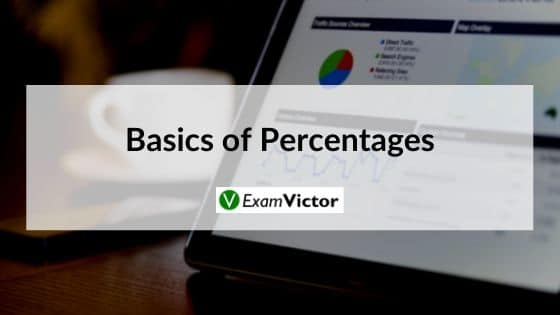# Basics of PercentagesLast Updated on Jan 30, 2020

Definition:
Percent implies “for every hundred” and the sign % is read as percentage and x % is read as x per cent. In other words, a fraction with denominator 100 is called a per cent. For example, 20 % means 20/100 (i.e. 20 parts from 100). This can also be written as 0.2.

Formula:
In order to calculate p % of q, use the formula:
(p/100) x q = (pxq)/100

Also remember: p % of q = q % of p

Examples:
1) 100% of 60 is 60 x (100/100) = 60
2) 50% of 60 is 50/100 × 60 = 30
3) 5% of 60 is 5/100 × 60 = 3

Example: 60 % of a number is 360. What is 99 % of the same number?
Solution: Let the number be n.
Given (60/100) ×n = 360 => n = 600
99 % of 600 = (99/100) × 600 = 594

Example: 50 % of a number is 360. What is 99 % of the same number?
Solution: Let the number be y.
Given (50/100) x q = 360
=> q = 720
99% of 720 = (99/100) x 720 = 712.80

Expressing One Quantity as a Per Cent with respect to the other:

To express a quantity as a per cent with respect to other quantity, the following formula is used:

percentage-basic-concept

Example: What percent is 60 of 240?
Solution: First write the given numbers in the fraction form:
60/240 = ¼
Multiply the numerator and denominator with 25 to make the denominator equal to 100
(1×25)/(4×25) = 25/100
25 percent or 25 per 100 is called as 25%

In order to convert a fraction into a percentage, multiply the fraction by 100 and put the “%” sign.

Example: ½ can be written as ½ x 100 = 50%.

To convert a percentage into a fraction, employ the reverse technique: divide the number by 100 and remove the “%” sign.

Example: 25% can be written as 25/100 = 1/4

To convert a percentage into a ratio, first convert the percentage into a fraction, and then the fraction into a ratio.

Example: Convert 25% into a ratio.
25% = 25/100 = ¼ = 1 : 4

##### Important Formulas for Percentage:

1) Percent implies “for every hundred”.
% is read as percentage and x % is read as x per cent.

2) To calculate p % of y
(p/100) x y = (p x y)/100

p % of y = y % of p

3) To find what percentage of x is y: y/x × 100

4) To calculate percentage change in value
Percentage change = {change/(initial value)} x 100

5) Percentage point change = Difference of two percentage figures

6) Increase N by S % = N( 1+ S/100 )

7) Decrease N by S % = N (1 – S/100)

8) If the value of an item goes up/down by x%, the percentage reduction/increment to be now made to bring it back to the original point is 100x/ (100 + x) %.

9) If A is x% more /less than B, then B is 100x/(100 + x) % less/more than A.

10) If the price of an item goes up/down by x %, then the quantity consumed should be reduced by 100x/ (100 + x)% so that the total expenditure remains the same.

11) Successive Percentage Change
If there are successive percentage increases of a % and b%, the effective percentage increase is:
{(a + b + (ab/100)}%

12) Product Stability Ratio:
A × B = P
If A is increased by a certain percentage, then B is required to be decreased by a certain percentage to keep the product P stable.

This video lays out the basics of Percentages. Understanding this topic well is necessary to solve questions that are asked in various competitive exams in the Logical Reasoning and Quantitative Aptitude section - applicable to CAT, XAT, MAT, SNAP, IIFT, CLAT, AILET, DU LLB, any other entrance exam as well.